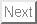Up: \$B%3%s%T%e!<%?!<\$O?t3X\$NK>1s6@(B \$BL58B\$O\$_\$(\$k\$+!)>ZL@\$O\$G\$-\$k\$+!)(B Previous: \$B%3%s%T%e!<%?!<\$O?t3X\$NK>1s6@(B \$BL58B\$O\$_\$(\$k\$+!)>ZL@\$O\$G\$-\$k\$+!)(B

# \$B\$3\$NJ8=q\$K\$D\$\$\$F(B...

\$B%3%s%T%e!<%?!<\$O?t3X\$NK>1s6@(B

-- \$BL58B\$O\$_\$(\$k\$+!)>ZL@\$O\$G\$-\$k\$+!)(B --

\$B\$3\$NJ8=q\$O(BLaTeX2HTML \$BK]Lu%W%m%0%i%`(B Version 99.1 release (March 30, 1999)

Copyright © 1993, 1994, 1995, 1996, Nikos Drakos, Computer Based Learning Unit, University of Leeds,
Copyright © 1997, 1998, 1999, Ross Moore, Mathematics Department, Macquarie University, Sydney.

\$B\$r(B\$BF|K\8l2=\$7\$?\$b\$N(B( 99.1 release + JA patch 3.0 (September 15, 1999) \$BHG(B)

Copyright © 1998, 1999, Kenshi Muto, Debian Project.

\$B\$rMQ\$\$\$F@8@.\$5\$l\$^\$7\$?!#(B

\$B%3%^%s%I9T\$O0J2<\$NDL\$j\$G\$7\$?!#(B:
latex2html abstract.tex.

\$BK]Lu\$O(B Masashi Katsurada \$B\$K\$h\$C\$F(B \$BJ?@.(B14\$BG/(B4\$B7n(B2\$BF|(B \$B\$KUp: \$B%3%s%T%e!<%?!<\$O?t3X\$NK>1s6@(B \$BL58B\$O\$_\$(\$k\$+!)>ZL@\$O\$G\$-\$k\$+!)(B Previous: \$B%3%s%T%e!<%?!<\$O?t3X\$NK>1s6@(B \$BL58B\$O\$_\$(\$k\$+!)>ZL@\$O\$G\$-\$k\$+!)(B